# How to Calculate and Solve for the Volume of a Spherical Segment | Nickzom Calculator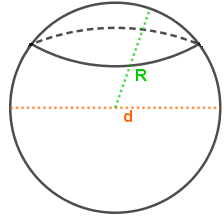The image above is a spherical segment.

To compute the volume of a spherical segment requires three essential parameters which are the radius of the spherical segment base (r1), radius of the spherical segment (r2) and height (h).

The formula for calculating the volume of the spherical segment:

V = πh(3r1² + 3r2² + h²)6

Where;
V = Volume of the spherical cap
r1 = Radius of the spherical segment base
r2 = Radius of the spherical segment base
h = Height of the spherical segment

Let’s solve an example;
Find the volume of a spherical segment when the radius of the spherical segment base (r1) is 7 cm, radius of the spherical segment base (r2) is 9 cm and a height of 20 cm.

This implies that;
r1 = Radius of the spherical segment base = 7 cm
r2 = Radius of the spherical segment base = 9 cm
h = Height of the spherical segment = 20 cm

V = πh(3r1² + 3r2² + h²)6
V = π x 20(3 x 7² + 3 x 9² + 20²)6
V = π x 20(3 x 49 + 3 x 81 + 400)6
V = π x 20(147 + 243 + 400)6
V = π x 20(790)6
V = π x 158006
V = 49643.66
V = 8273.9

Therefore, the volume of the spherical segment is 8273.9 cm3.

Nickzom Calculator – The Calculator Encyclopedia is capable of calculating the volume of the spherical segment.

To get the answer and workings of the volume of the spherical segment using the Nickzom Calculator – The Calculator Encyclopedia. First, you need to obtain the app.

You can get this app via any of these means:

To get access to the professional version via web, you need to register and subscribe for NGN 1,500 per annum to have utter access to all functionalities.
You can also try the demo version via https://www.nickzom.org/calculator

Once, you have obtained the calculator encyclopedia app, proceed to the Calculator Map, then click on Mensuration under the Mathematics section.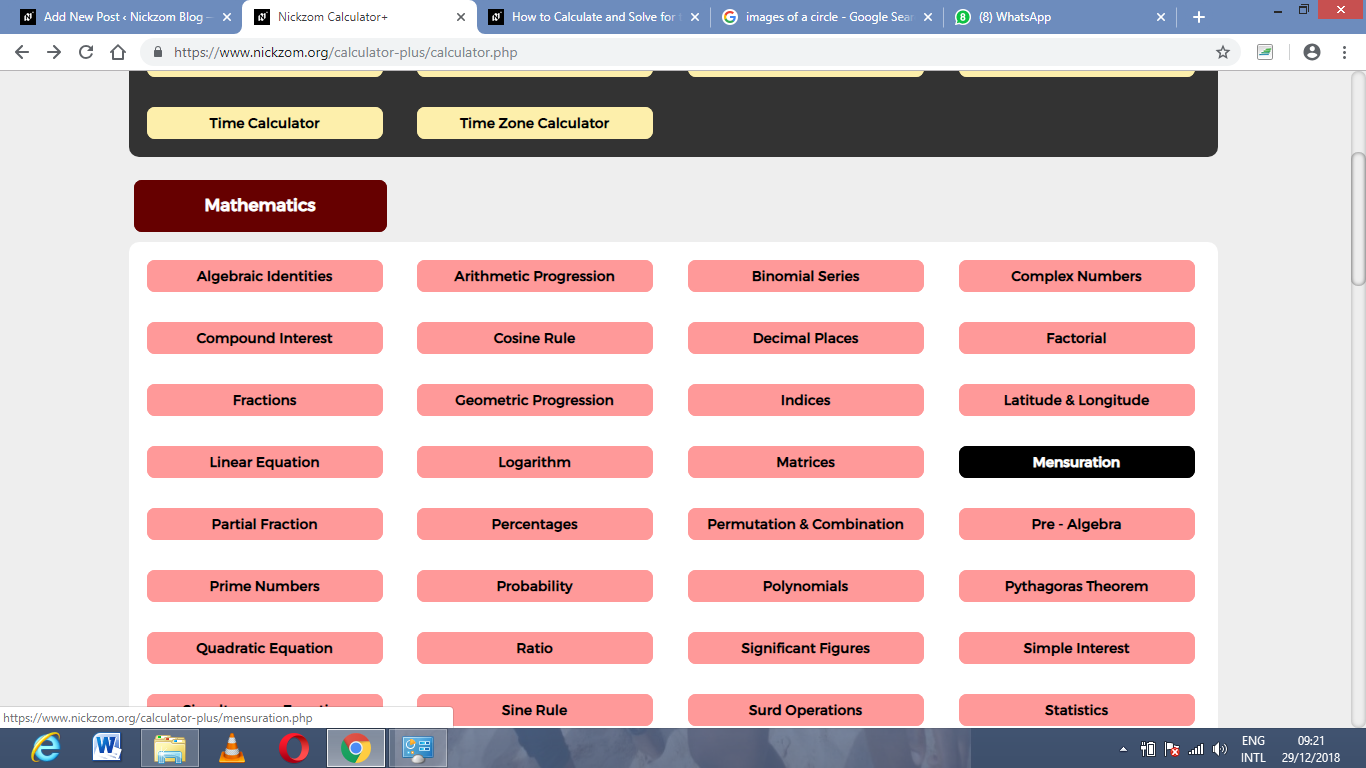Now, click on Volume of the spherical segment under Mensuration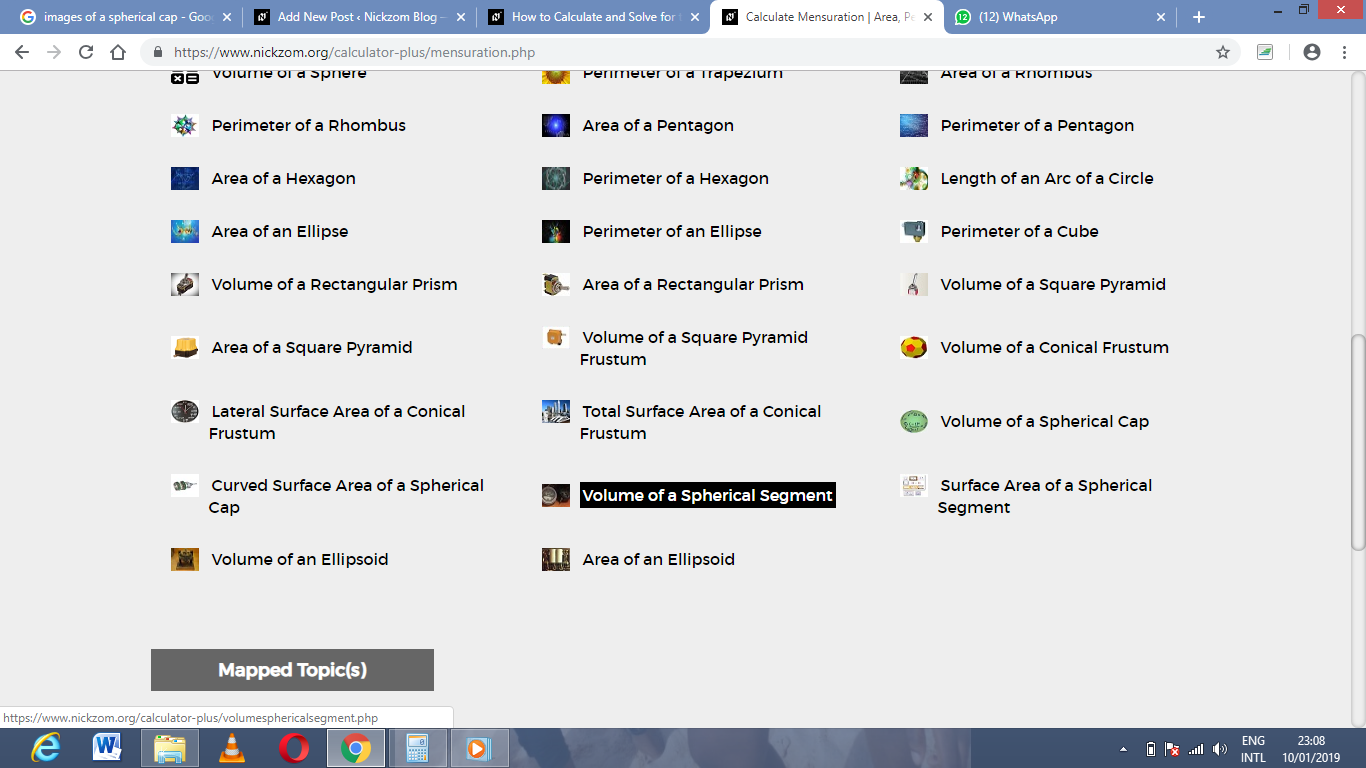The screenshot below displays the page or activity to enter your values, to get the answer for the volume of the spherical segment according to the respective parameters which are the radius of the spherical segment base (r1), radius of the spherical segment (r2) and height (h).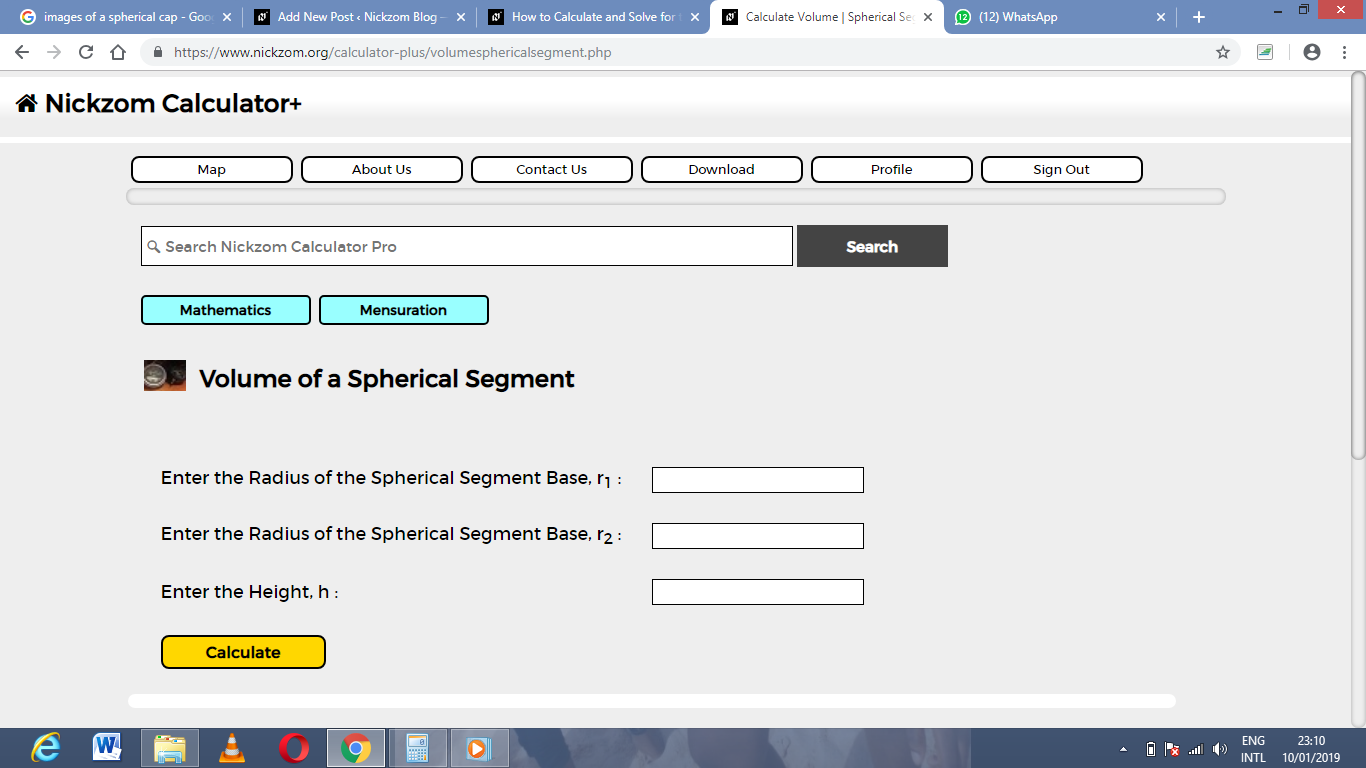Now, enter the values appropriately and accordingly for the parameters as required by the example above where the radius of the spherical segment base (r1) is 7 cm, radius of the spherical segment (r2) is 9 cm and height (h) is 20 cm.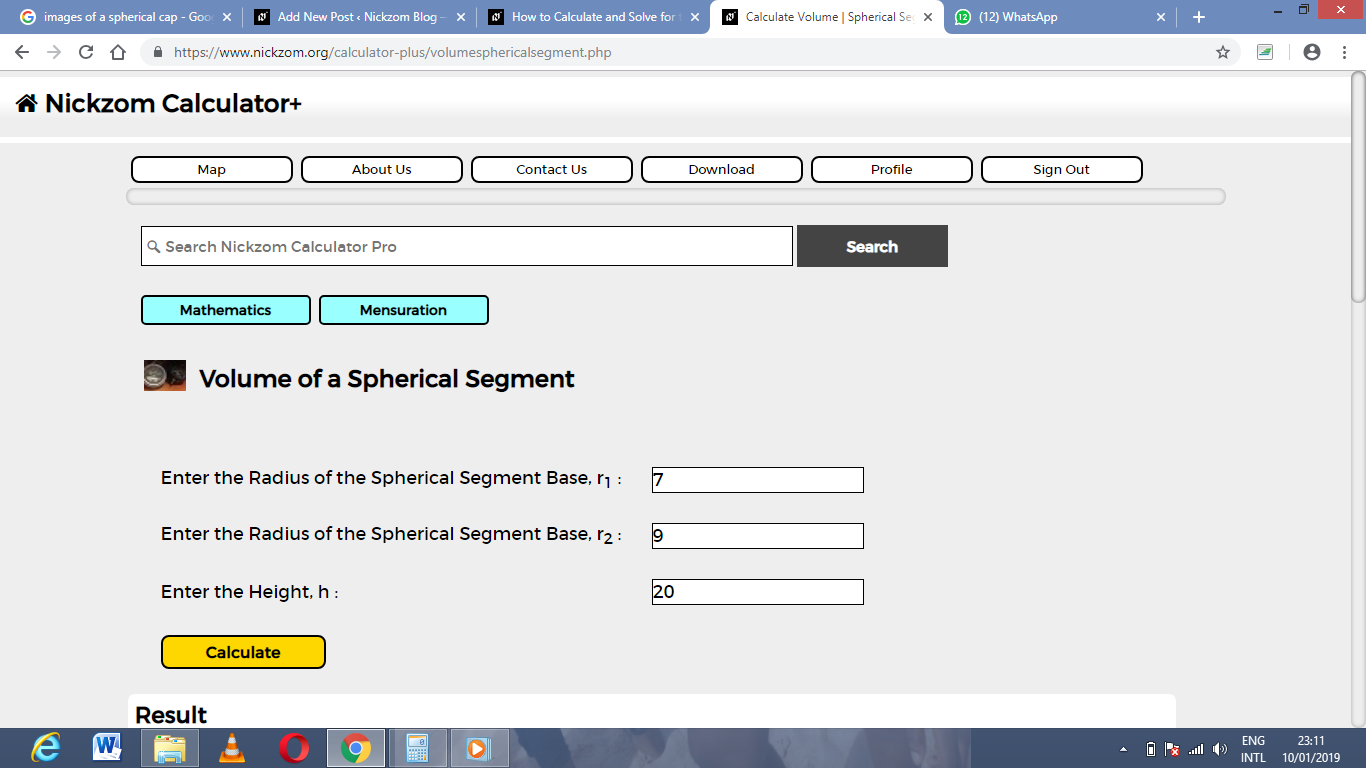Finally, Click on Calculate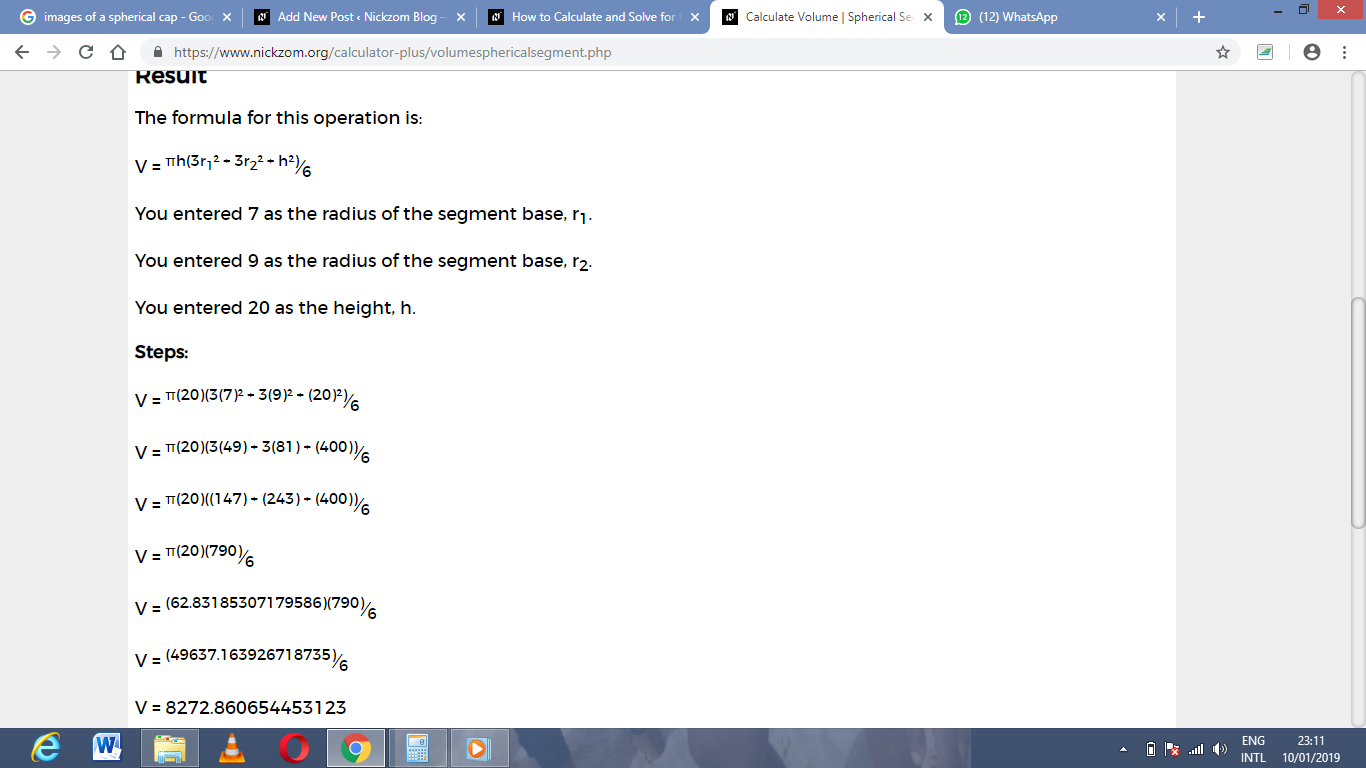As you can see from the screenshot above, Nickzom Calculator – The Calculator Encyclopedia solves for the Volume of a spherical segment and presents the formula, workings and steps too.